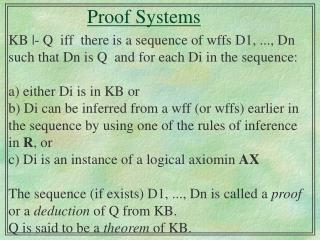# Proof Systems - PowerPoint PPT PresentationDownload PresentationProof Systems

Proof Systems
Download Presentation## Proof Systems

- - - - - - - - - - - - - - - - - - - - - - - - - - - E N D - - - - - - - - - - - - - - - - - - - - - - - - - - -
##### Presentation Transcript

1. Proof Systems KB |- Q iff there is a sequence of wffs D1, ..., Dn such that Dn is Q and for each Di in the sequence: a) either Di is in KB or b) Di can be inferred from a wff (or wffs) earlier in the sequence by using one of the rules of inference in R, or c) Di is an instance of a logical axiomin AX The sequence (if exists) D1, ..., Dn is called a proof or a deduction of Q from KB. Q is said to be a theorem of KB. KB |- Q : a) by the definition of entailment:

2. What is soundness? For every KB and Q, if KB |- Q then KB |= Q Informally, a proof system is sound if it only generates entailed wffs (every positive answer is correct) (remember that the semantical system is the reference) A sound proof system is truth-preserving: any model for the original set of wffs (KB) is also a model for the derived set of wffs (Q).

3. Completeness • One other question we can ask is whether using our proof system we can generate all of the entailed wffs (the system can give all the correct answers) • If we are able to do so, we say that our inference procedure is complete: For every KB and Q, if KB |= Q then KB |- Q Equivalent form: if KB |/- Q then KB ||/- Q

4. Complexity • Truth-tables are exponential in the number of atoms: 2n interpretations • [Cook 71] showed that Satisfiability is a NP-complete problem. • But in many cases answers can be found very quickly (Horn-Sat is solvable linear time) • in fact really hard problems are quite rare (see hw).

5. Proof Systems Several proof systems in the literature: • Resolution (the only one we will study) SLD resolution - basis of PROLOG • Tableaux • Natural Deduction • Sequent Calculus (Gentzen) • Axiomatic (Hilbert)

6. Clauses as wffs • More adequate for computation - canonical form • A literal is either an atom (positive literal) or the negation of an atom (negative literal). • A clause is a disjunction of literals; the empty clause is equivalent to False. • A wwf is in Conjunctive Normal Form (CNF) iff it is a set of clauses (the set is abreviating the conjunction of all the clauses).

7. Converting arbitrary wffs to CNF • Eliminate implications: A  B becomesA  B • Move  inwards: • Apply De Morgan’s : (A v B) becomes (A B) (A  B) becomes (A v B) • Apply double negation rule:   A becomes A

8. Converting arbitrary wffs to CNF Distribute  over v : (A  B) v C becomes (A v C)  (B v C) Flatten nested conjunctions and disjunctions: (A v B) v C becomes (A v B v C) (A  B)  C becomes (A  B  C) At this point we have a conjunction of clauses; We must have a set of clauses! separate the conjuncts

9. Important Theorem • Let S be a set of wffs and S’ the set of clauses obtained by converting S to CNF. • In Propositional Logic S and S’ are equivalent; but in FOL they are not equivalent in general • But in both logics we have: S is unsatisfiable iff S’ is unsatisfiable. • Therefore, KB |= Q iff S = KB U { Q}is unsat iff S’ is unsat

10. Resolution System • Language: Clauses • Logical Axioms: AX = { } • Inference Rules: R = {Resolution} Notice that since the language is clausal, resolution is applied only to clauses: P1 v ... v Pi v ... v Pn , Q1 v ... v  Pi v ... v Qm --------------------------------------------------------- P1v...vPi-1vPi+1v...vPn vQ1v...vQj1vQj+1v...vQm The conclusion is called the resolvent

11. Resolution System • Soundness Since its only rule is resolution and there are no logical axioms, it is easy to show that the resolution system is sound: show the soundness of the resolution inference rule (show by truth-table that the premisses entail the conclusion) and then show by induction on the length of a proof that if S’ |- False then S’ ||= False.

12. Resolution System • Completeness: Resolution is not complete P , R |= P V R but P , R |/- P V R But Resolution is Refutation Complete: Let S’ = CNF(KB U { Q}) If KB |= Q then S’ |- False P , R,  P,  R |- False

13. Resolution System To answer if KB |= Q: • Convert S = KB U { Q} into S’ = CNF(S) convert each formula of S into clauses • Iteratively apply resolution to the clauses in S’ and add the results to S’ either until there are no more resolvents that can be added or until the empty clause is produced.

14. Refinement Strategies • The procedure described above is inefficient because some resolutions need not be performed at all (are irrelevant). • Refinement strategies disallows certain kinds of resolutions to take place. • Linear resolution with initial set of support

15. Proof as a search task • State representation: a set of wffs (considered to to be true) • Operators: inference rules • Start state: an initial set of wffs (what is initially considered to to be true) • Goal state: the wff to prove is in our state’s set of known wffs